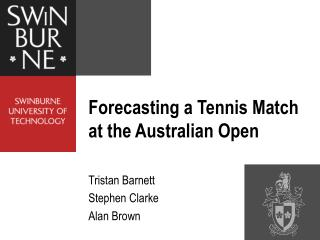DownloadDownload PresentationForecasting a Tennis Match at the Australian Open

# Forecasting a Tennis Match at the Australian Open

Download Presentation## Forecasting a Tennis Match at the Australian Open

- - - - - - - - - - - - - - - - - - - - - - - - - - - E N D - - - - - - - - - - - - - - - - - - - - - - - - - - -
##### Presentation Transcript

1. Forecasting a Tennis Match at the Australian Open Tristan Barnett Stephen Clarke Alan Brown

2. Introduction • Match Predictions Markov Chain Model Collecting Data Exponential Smoothing Combining Player Statistics • Real Time Predictions Combining Sheets from Markov Chain Model Bayesian Updating Rule Excel Computer Demonstration

3. Markov Chain Model • Modelling a game of tennis Recurrence Formula: P(a,b) = pP(a+1,b) + (1-p)P(a,b+1) Boundary Conditions: P(a,b) = 1if a=4, b ≤ 2 P(a,b) = 0if b=4, a ≤ 2 where for player A: p = probability of winning a point on serve P(a,b) = conditional probability of winning the game when the score is (a,b)

4. Markov Chain Model Table 1:The conditional probabilities of player A winning the game from various score lines for p = 0.6 • Similarly sheet for player B serving sheets for a set (from sheets of a game) sheet for a match (from sheets of a set)

5. Collecting Data The ATP tour matchfacts: http://www.atptennis.com/en/media/rankings/matchfacts.pdf

6. Collecting Data fi = ai bi + (1 - ai ) ci gi = aav di + ( 1 - aav ) ei where the percentage for player i : fi = points won on serve gi = points won on return ai = 1st serves in play bi = points won on 1st serve ci = points won on 2nd serve di = points won on return of 1st serve ei = points won on return of 2nd serve where the percentage for average player on the ATP tour: aav = 1st serves in play= 58.7%

7. Exponential Smoothing Fit = Fit-1 + [ 1 - ( 1 – α)n ][ fit - Fit-1 ] Git = Git-1 + [ 1 - ( 1 – α)n ] [ git - Git-1 ] where: For player i at period t Fit = smoothed average of the percentage of points won on serve after observing fitGit= smoothed average of the percentage of points won on return of serve after observing git Initialised for average ATP tour player Fi0 = the ATP average of percentage of points won on serve Gi0 = the ATP average of percentage of points won on return of serve n = number of matches played since period t-1 α =smoothing constant • When n=1, [1-(1-α)n] = α, as expected • When n becomes large, [1-(1-α)n] → 1, as expected

8. Combining Player Statistics fij = ft + ( fi - fav ) - ( gj - gav ) • gji = gt + ( gj - gav ) - ( fi - fav ) • where: • For thecombined player statistics • fij= percentage of points won on serve for player i against player j • gji =percentage points won on return for player j against player I • For thetournament averages • ft = percentage of points won on serve • gt = percentage of points won on return of serve • For theATP tour averages • fav = percentage of points won on serve • gav = percentage of points won on return of serve • Since ft + gt = 1, fij + gji = 1 for all i,j as required

9. Combining Sheets The equation for theprobability of player A winning a best-of-5 set match from (e,f) in sets, (c,d) in games, (a,b) in points, player A serving. P''(a,b:c,d:e,f ) = P(a,b) P'B(c+1,d) P''(e+1,f ) + P(a,b) [1-P'B(c+1,d)] P''(e,f+1) + [1-P(a,b)] P'B(c,d+1) P''(e+1,f ) + [1-P(a,b)] [1-P'B(c,d+1)] P''(e,f+1) where for player A : P''(a,b:c,d:e,f ) = probability of winning the match from (a,b:c,d:e,f ) P'B(c,d) = probability of winning the set from (c,d) when player B is serving P''(e,f ) = probability of winning the match from (e,f )

10. Bayesian Updating Rule where: θti= updated percentage of points won on serve at time t for player i μi =initial percentage of points won on serve for player i φti= actual percentage of points won on serve at time t for player i n = number of points played M = expected points to be played When n=0, θ0i= μi as expected When M →0,θti→ φti

11. Computer Demonstration ISF3.XLS 2003 Australian Open Quarter Final El Aynaoui versus Roddick

12. Computer Demonstration ISF4.XLS End of 1st set where: = game to El Aynaoui = game to Roddick = set to El Aynaoui

13. Computer Demonstration End of match where: = game to El Aynaoui by breaking serve = game to Roddick by breaking serve = set to El Aynaoui = set to Roddick